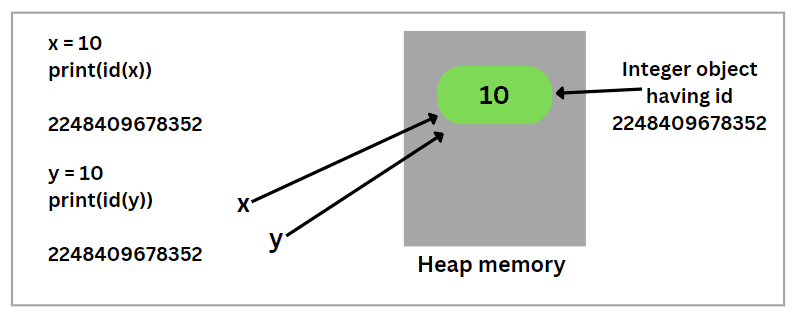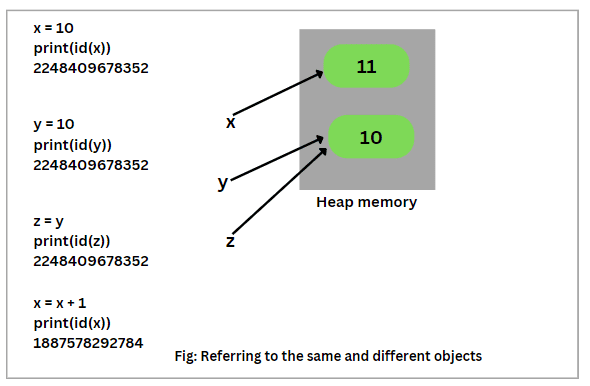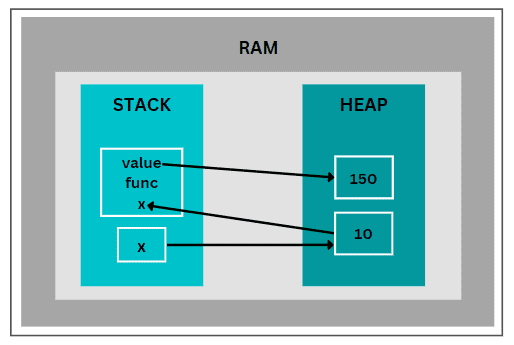# Memory Management in Python with Example

Memory management in Python is the process by which the computer system reserves a part or complete section of memory for the process and execution of programs.

It allows the application programs to read and write data. We know that there is an enormous size of memory in the computer system.

It is essential to allocate some free space in the memory and make it available for the execution of the program.

This way of allocating (i.e. providing) memory for the execution of a program is called memory allocation.

Also, when data is no longer in use, must be removed or erased from the memory after being execution of code. Knowledge of memory management helps developers to write the efficient code.

## How does Python Manage Memory?

In C and C++, we may need to allocate and de-allocate memory dynamically during runtime of a program. Dynamic memory allocation is a term for it.

Basically, dynamic memory allocation is the allocation of the memory at runtime as the program executes. For example, to allocate memory, we can use malloc() function and to de-allocate the memory, we can use the free() function.

However, Python handles memory allocation and de-allocation at runtime automatically. We do not need to allocate while creating objects or de-allocate memory when deleting objects.

Python interpreter is the responsible for dynamically allocation and de-allocation of the memory. In other words, the Python interpreter takes care of memory management.

In Python, everything is considered as an object. For example, numbers, strings, functions, dictionary, lists, modules, and user-defined classes are considered objects in Python.

We can access them with the help of identifier. Python’s memory manager allocates the memory to the objects as per requirement. It uses a private heap space for memory management, which is not accessible by the programmer.

All the Python objects and data structures are allocated in this heap space. The memory manager through Python API functions internally manages this private heap space for Python objects.

Python has also an in-built garbage collector, which recycles all the unused memory so that free space is available in heap memory.

## Python Memory Management Example

In this section, let’s have a look at how things work. Suppose we assign a value of 10 to a variable x:

```x = 10
```

Here, 10 is an integer object in memory and “x” is a reference variable pointing to this integer object. Look at the below example code.

```x = 10
print(id(x))
```
```Output:
2248409678352
```

In this example, the id() function generates a unique identification number for an object. This unique identification number is an integer value which will remain unique and constant for the Python object during its lifespan, as shown in the below figure. The id for integer object 10 is 2248409678352.Two objects with non-overlapping lifetimes can have the identical id value. Let’s understand it with an example. Suppose we assign the same value 10 to a new variable y. Look at the following code snippet.

```x = 10
print(id(x))
y = 10
print(id(y))
```
```Output:
2070047162896
2070047162896
```

As you can observe in the output, both reference variables x and y are pointing to the same integer object 10 because of the same unique identification number. Look at the below figure that x and y have reference to the same object.Now, consider the following example code below.

```x = 10
print(id(x))

y = 10
print(id(y))

z = y
print(id(z))
```
```Output:
2070047162896
2070047162896
2070047162896
```

As you can see in the output, the id() function generated the same identification number for the integer object 10. Therefore, z is also pointing to the same object.Now, suppose we are performing the following operation in the above code.

```x = 10
print(id(x))
y = 10
print(id(y))

z = y
print(id(z))
x = x + 1
print(id(x))
```
```Output:
1887578292752
1887578292752
1887578292752
1887578292784
```

Now, as you can see in the output, the id() function has generated the different identification number for this operation x = x + 1. Therefore, x is not equal to 11 and now refers to the different integer object 11.## Stack and Heap Memory in RAM

The memory management in Python is divided into two parts mainly.

1. Stack memory:
• In the stack memory, all methods are executed.
• References to Python objects in the heap memory are produced in the stack memory.
2. Heap memory:
• All Python objects are created in the heap memory.

Let’s have a look at how memory management in Python works with the help of an example. Look at the following piece of program code.

```def func(x):
value = (x + 5) * 10
return value
x = 10
final_value = func(x)
print("Final value = ", final_value)
```
```Output:
Final value =  150
```

Now, let’s understand how this code is working. The execution of program code begins from the main which in this case is:

```x = 10
final_value = func(x)
print("Final value = ", final_value)
```

Step 1: Execute x = 10

This statement creates an integer object 10 in the heap memory and the reference variable x is created in the main stack memory that is pointing to this integer object, as shown in the below figure.Step 2: Execute final_value = func(x)

This statement will call func(x).

```def func(x):
value = (x + 5) * 10
return value
```

In order to execute func(x), Python interpreter adds a new stack frame in the memory. Till the time, func(x) is being executed, the interpreter put on hold the value 10 referenced by x of the lower stack frame. The integer value 10 is passed as an argument to this function.Now, value = (x + 5) * 10 = (10 + 5) * 10 = 150 (refer below figure). The func() function assigns the value of 150 to the value.Now, the func() function will return the value 150, and the interpreter will assign it to the final_value in the main stack memory. Look at the following figure below.Here, it is important to note that the management of heap memory in Python is performed by the interpreter itself and the programmer has no control over it.

Python memory manager through the Python API functions, allocates the memory in heap space for Python objects and other internal buffers on demand.

In this tutorial, we have told about memory management in Python with example and lots of diagrams. Hope that you will have understood how does the Python interpreter handles memory management automatically.